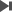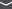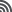UAB Digital Repository of Documents 165 records found  1 - 10jump to record: Search took 0.01 seconds.
1.
 27 p, 5.2 MB Risk analysis through the half-normal distribution / Bosch-Badia, Maria-Teresa (Universitat de Girona. Departament d'Economia) ; Montllor i Serrats, Joan (Universitat Autònoma de Barcelona. Departament d'Empresa) ; Tarrazón Rodón, Ma. Antonia (Universitat Autònoma de Barcelona. Departament d'Empresa) We study the applicability of the half-normal distribution to the probability-severity risk analysis traditionally performed through risk matrices and continuous probability-consequence diagrams (CPCDs). [...] 2020 -10.3390/math8112080 Mathematics, Vol. 8 Núm. 11 (2020) , p. 2080
2.
 20 p, 10.5 MB Mathematical modeling projects oriented towards social impact as generators of learning opportunities : a case study / Albarracín Gordo, Lluís (Universitat Autònoma de Barcelona. Departament de Didàctica de la Matemàtica i de les Ciències Experimentals) ; Gorgorió Solà, Núria (Universitat Autònoma de Barcelona. Departament de Didàctica de la Matemàtica i de les Ciències Experimentals) This paper presents a case study carried out at an elementary school that led to a characterization of mathematical modeling projects aimed at generating social impact. It shows their potential as generators of mathematical learning opportunities. [...] 2020 -10.3390/math8112034 Mathematics, Vol. 8, núm. 11 (2020) , p. e2034
3.
 20 p, 2.7 MB Graph-based problem explorer : a software tool to support algorithm design learning while solving the salesperson problem / Hernàndez i Sabaté, Aura (Universitat Autònoma de Barcelona. Departament de Ciències de la Computació) ; Albarracín Gordo, Lluís (Universitat Autònoma de Barcelona. Departament de Didàctica de la Matemàtica i de les Ciències Experimentals) ; Sánchez Pujadas, Francisco Javier (Universitat Autònoma de Barcelona. Departament de Ciències de la Computació) In this article, we present a sequence of activities in the form of a project in order to promote learning on design and analysis of algorithms. The project is based on the resolution of a real problem, the salesperson problem, and it is theoretically grounded on the fundamentals of mathematical modelling. [...] 2020 -10.3390/math8091595 Mathematics, Vol. 8, núm. 9 (2020) , p. e1595
4.
 30 p, 951.8 KB On the configurations of centers of planar Hamiltonian Kolmogorov cubic polynomial differential systems / Llibre, Jaume (Universitat Autònoma de Barcelona. Departament de Matemàtiques) ; Xiao, Dongmei (Shanghai Jiao Tong University. School of Mathematical Sciences (China)) We study the kind of centers that Hamiltonian Kolmogorov cubic polynomial differential systems can exhibit. Moreover, we analyze the possible configurations of these centers with respect to the invariant coordinate axes, and obtain that the real algebraic curve xy(a+bx+cy+dx2+exy+fy2)=h has at most four families of level ovals in R2 for all real parameters a,b,c,d,e,f and h. 2020 -10.2140/pjm.2020.306.611 Pacific Journal of Mathematics, Vol. 306, Issue 2 (2020) , p. 611-644
5.
 12 p, 630.6 KB On the period function in a class of generalized Lotka-Volterra systems / Villadelprat, Jordi (Universitat de Barcelona. Departament de Matemàtica Aplicada i Anàlisi) In this note, motivated by the recent results of Wang et al. [Wang et al. , Local bifurcations of critical periods in a generalized 2D LV system, Appl. Math. Comput. 214 (2009) 17-25], we study the behaviour of the period function of the center at the point (1,1) of the planar differential system {u' = up(1−vq),v'= μvq(up−1), where p, q, μ ∈ R with pq > 0 and μ > 0. [...] 2010 -10.1016/j.amc.2010.03.025 Applied Mathematics and Computation, Vol. 216, Issue 7 (June 2010) , p. 1956-1964
6.
 14 p, 290.3 KB On the zero-Hopf bifurcation of the Lotka-Volterra systems in R3 / Han, Maoan (Shanghai Normal University. Department of Mathematics (China)) ; Llibre, Jaume (Universitat Autònoma de Barcelona. Departament de Matemàtiques) ; Tian, Yun (Shanghai Normal University. Department of Mathematics (China)) Here we study the Lotka-Volterra systems in R3, i. e. the differential systems of the form dxi/dt = xi(ri - Σ3j=1 aijxj), i = 1, 2, 3. It is known that some of these differential systems can have at least four periodic orbits bifurcating from one of their equilibrium points. [...] 2020 -10.3390/math8071137 Mathematics, Vol. 8, Issue 7 (July 2020) , art. 1137 2 documents
7.
 A bound on the number of rationally invisible repelling orbits / Benini, Anna Miriam (Università di Parma. Dipartimento di Scienze Matematiche Fisiche e Informatiche (Italy)) ; Fagella Rabionet, Núria (Universitat de Barcelona. Departament de Matemàtiques i Informàtica) We consider entire transcendental maps with bounded set of singular values such that periodic rays exist and land. For such maps, we prove a refined version of the Fatou-Shishikura inequality which takes into account rationally invisible periodic orbits, that is, repelling cycles which are not landing points of any periodic ray. [...] 2020 -10.1016/j.aim.2020.107214 Advances in Mathematics, Vol. 370 (August 2020) , art. 107214
8.
 14 p, 596.0 KB Crossing limit cycles of planar piecewise linear Hamiltonian systems without equilibrium points / Benterki, Rebiha (Université Mohamed El Bachir El Ibrahimi. Département de Mathématiques (Algeria)) ; Llibre, Jaume (Universitat Autònoma de Barcelona. Departament de Matemàtiques) In this paper, we study the existence of limit cycles of planar piecewise linear Hamiltonian systems without equilibrium points. Firstly, we prove that if these systems are separated by a parabola, they can have at most two crossing limit cycles, and if they are separated by a hyperbola or an ellipse, they can have at most three crossing limit cycles. [...] 2020 -10.3390/MATH8050755 Mathematics, Vol. 8, Issue 5 (May 2020) , art. 755 2 documents
9.
 10 p, 652.9 KB Limit cycles for a class of third-order differential equations / Llibre, Jaume (Universitat Autònoma de Barcelona. Departament de Matemàtiques) ; Yu, Jiang (Shanghai Jiaotong University. Department of Mathematics (China)) ; Zhang, Xiang (Shanghai Jiaotong University. Department of Mathematics (China)) In this paper we study the limit cycles of the third-order differential equation . . . x − μẍ + ẋ − μx = εF(x, ẋ , ẍ, t) where μ ≠ 0, ε is small enough and F Є C2 is a 2π-periodic function of variable t. 2010 -10.1216/RMJ-2010-40-2-581 The Rocky Mountain Journal of Mathematics, Vol. 40, Issue 2 (2010) , p. 581-594 2 documents
10.
 14 p, 663.8 KB On Poncelet's maps / Cimà, Anna (Universitat Autònoma de Barcelona. Departament de Matemàtiques) ; Gasull i Embid, Armengol (Universitat Autònoma de Barcelona. Departament de Matemàtiques) ; Mañosa Fernández, Víctor 1971- (Universitat Politècnica de Catalunya. Departament de Matemàtica Aplicada III) Given two ellipses, one surrounding the other one, Poncelet introduced a map P from the exterior one to itself by using the tangent lines to the interior ellipse. This procedure can be extended to any two smooth, nested and convex ovals and we call these types of maps, Poncelet's maps. [...] 2010 -10.1016/j.camwa.2010.06.027 Computers and Mathematics with Applications, Vol. 60, Issue 5 (September 2010) , p. 1457-1464

UAB Digital Repository of Documents : 165 records found   1 - 10jump to record:
Interested in being notified about new results for this query?
Set up a personalemail alert or subscribe to theRSS feed.